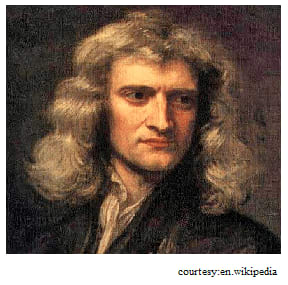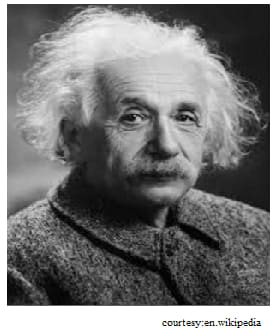# What is nature of gravity?Gravity is the king of all forces. It has a big role in the creation of this universe. Sir Issac Newton is the first thing we remember when we talk about gravity. Newton has done immense contribution to the world by discovering about gravity and gravitational force. Most of the forces or scientific calculations are all being done based on gravitational force. Have you ever imagined how the world would be without gravity? The gravity is the power holding all the creatures in this world. How would plants grow? How would you do your daily activities? It feels like life existing possibilities on this earth would become less without gravity. The intensity of gravitational pull differs from planet to planet.

How will the gravity ascend from the earth? How it happens in other planet bodies?

Gravity or the gravitational force is the natural phenomenon which holds or attracts different particles. Any particle with mass is brought towards or together by gravitational force. It may be planets, sub atomic particles, stars anything and everything which possesses mass will experience gravitational effect. More the mass of the object more will be the gravitational force. This gravitational pull is what keeps the object intact with the ground, the objects thrown up returns back.

You know the story behind the discovery of gravity. Apple fell on Newton when he was resting under a tree. Actually when apple fell off the tree, Newton wondered what is that which made the apple fall to ground. At what speed it reached ground? That is when he started analysing the causes of it. He understood that any object will be attracted towards ground due to a pull called gravitational pull. The speed at which the object is landed on the ground depends on the mass of the object.

Gravity is most precisely explained by Einstein in 1915 in his general theory of relativity. He described gravity as the force that happens due to the space which is curved or wrapped around with mass. He considered it as a consequence of curvature rather than a force. There were quite a number of experiments proved that Einstein was right. But there was few questions which he could not answer. However for most of the experiments the Newton’s law gravitational force is most approximated.

Newton postulated that gravitational force has an infinite range. The force of attraction between objects weakens as the inverse square of the distance between the objects. And the gravitational force is mathematically expressed as proportional to product of masses and inversely proportional to the square of the distance between the bodies.

Earth’s gravityEarth’s gravity is denoted by ‘g’. Where g=9.80665. So the strength of the gravitational pull is numerically equal to the acceleration of the body under its influence. The acceleration rate of object falling on the earth differs according to the surface and altitude etc. The gravity possessed by sub atomic particles are negligible. The gravity is vector sum of two forces ie gravitational force and the centrifugal force. The gravitational force differs with latitude. It varies from equator to poles. The force of gravity at equator is 9.780 and increases to 9.832 at the poles.

The properties of gravity show how this force is so essential.

• Time doesn’t affect gravity. So gravity will not change with time.
• Gravity is the force which only attracts, whereas some other forces have both attractive and repulsive force.
• The force of gravity declines with distance.
• Attraction of gravity depends on the mass of object but not on its composition.
• There is no antigravity. The gravity cannot be shielded.